Next: Self-inductance Up: Magnetic induction Previous: Introduction

## Inductance

We have learned about e.m.f., resistance, and capacitance. Let us now investigate inductance. Electrical engineers like to reduce all pieces of electrical apparatus to an equivalent circuit consisting only of e.m.f. sources (e.g., batteries), inductors, capacitors, and resistors. Clearly, once we understand inductors, we shall be ready to apply the laws of electromagnetism to electrical circuits.

Consider two stationary loops of wire, labeled 1 and 2. Let us run a steady current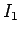around the first loop to produce a magnetic field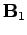. Some of the field lines ofwill pass through the second loop. Letbe the flux ofthrough loop 2:(896)

where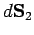is a surface element of loop 2. This flux is generally quite difficult to calculate exactly (unless the two loops have a particularly simple geometry). However, we can infer from the Biot-Savart law,(897)

that the magnitude ofis proportional to the current. This is ultimately a consequence of the linearity of Maxwell's equations. Here,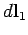is a line element of loop 1 located at position vector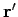. It follows that the fluxmust also be proportional to. Thus, we can write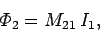(898)

where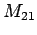is the constant of proportionality. This constant is called the mutual inductance of the two loops.

Let us write the magnetic fieldin terms of a vector potential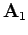, so that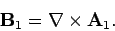(899)

It follows from Stokes' theorem that(900)

where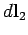is a line element of loop 2. However, we know that(901)

The above equation is just a special case of the more general law,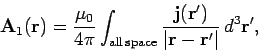(902)

for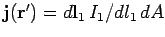and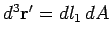, where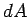is the cross-sectional area of loop 1. Thus,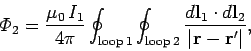(903)

where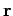is now the position vector of the line elementof loop 2. The above equation implies that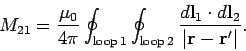(904)

In fact, mutual inductances are rarely worked out from first principles--it is usually too difficult. However, the above formula tells us two important things. Firstly, the mutual inductance of two loops is a purely geometric quantity, having to do with the sizes, shapes, and relative orientations of the loops. Secondly, the integral is unchanged if we switch the roles of loops 1 and 2. In other words,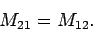(905)

In fact, we can drop the subscripts, and just call these quantities. This is a rather surprising result. It implies that no matter what the shapes and relative positions of the two loops, the magnetic flux through loop 2 when we run a current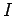around loop 1 is exactly the same as the flux through loop 1 when we send the same current around loop 2.

We have seen that a currentflowing around some loop, 1, generates a magnetic flux linking some other loop, 2. However, flux is also generated through the first loop. As before, the magnetic field, and, therefore, the flux, is proportional to the current, so we can write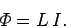(906)

The constant of proportionality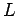is called the self-inductance. Likeit only depends on the geometry of the loop.

Inductance is measured in S.I. units called henries (H): 1 henry is 1 volt-second per ampere. The henry, like the farad, is a rather unwieldy unit, since most real-life inductors have a inductances of order a micro-henry.Next: Self-inductance Up: Magnetic induction Previous: Introduction
Richard Fitzpatrick 2006-02-02